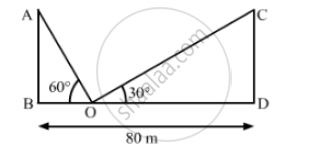# Two Poles of Equal Heights Are Standing Opposite to Each Other on Either Side of the Road Which is 80m Wide, from a Point P Between Them on the Road Find the Height of Each Pole and Distance. - Mathematics

Two poles of equal heights are standing opposite to each other on either side of the road which is 80m wide, From a point P between them on the road, the angle of elevation of the top of one pole is 60 and the angle of depression from the top of another pole at P is 30 . Find the height of each pole and distance of the point P from the poles.

#### SolutionLet AB and CD be the equal poles; and BD be the width of the road.
We have,
∠AOB = 60° and ∠COD = 60°
In ΔAOB,

tan 60° = (AB)/(BO)

⇒ sqrt(3) = (AB)/ (BO)

⇒ BO = (AB) / sqrt(3)

Also, in ΔCOD,

tan 30° = (CD)/(DO)

⇒  1/ sqrt(3) = (CD)/(DO)

⇒ DO = sqrt(3)CD

As , BD = 80

⇒ BO +DO = 80

⇒ (AB) /sqrt(3) + sqrt(3) CD = 80

⇒ (AB) /sqrt(3) + sqrt(3) AB = 80           (Given : AB - CD)

⇒AB (1/sqrt(3) + sqrt(3) ) = 80

⇒ AB ((1+3)/sqrt(3) )=80

⇒ AB (4/ sqrt(3) ) =80

⇒ AB = (80+sqrt(3))/4

⇒ AB = 20 sqrt(3) m

Also , BO = (AB)/ sqrt(3) = (20sqrt(3))/sqrt(3) = 20m

So, DO = 80 - 20 = 60m
Hence, the height of each pole is 20 sqrt(3)m and point P is at a distance of 20 m from left pole ad 60 m from right pole.

Concept: Heights and Distances
Is there an error in this question or solution?
Chapter 14: Height and Distance - Exercises

#### APPEARS IN

RS Aggarwal Secondary School Class 10 Maths
Chapter 14 Height and Distance
Exercises | Q 11

Share